# IBPS Clerk Prelims Reasoning Question Paper 2021

IBPS conducts various banks exams like IBPS Clerk, IBPS PO, IBPS SO etc. to encourage the Recruitment of young talent in various National Public Sector Banks. These exams are conducted to fill 4-5K vacancies every year.

When we talk about the IBPS Clerk exam, it is conducted for the Recruitment of “Clerical Grade” in the various Public sector banks of India. IBPS Clerk Notification 2022 will be released in July 2022,  It will be a golden opportunity for those, who want to serve in Public Sector Banks.

In this article, we have provided “Memory-Based Reasoning Paper of IBPS Clerk Prelims 2021”, to give you an idea of the current pattern of IBPS Clerk and score good marks in the exam.

For more practice read our other articles related to it.

### Directions (1-3): Study the information carefully and answer the questions given below.

A certain number of persons are sitting in a row facing north. Only two persons sit between A and E. C sits second to the left of E. B sits fourth to the right of F. D sits to the right of B. A sits fourth to the left of F and only two persons sit left of A. Not more than five persons sit between D and E. Eight persons sit between D and G, who sits at one of the extreme ends.

### Solution(1-3):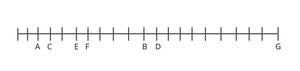Q1. How many people sit in the row?

(a) 22

(b) 21

(c) 19

(d) 24

(e) 18

Solution: b

21 persons sit in the row.

Q2. How many persons are sitting between F and E?

(a) One

(b) Two

(c) None

(d) Three

(e) More than three

Solution: c

No person is sitting between F and E.

Q3. Who sits 5th to the left of D?

(a) F

(b) C

(c) A

(d) B

(e) More than thirteen

Solution: a

F sits 5th to the left of D.

Directions (4-8): In these questions, the relationship between different elements is shown in the statements. The statements are followed by conclusions. Give answer:

Q4. Statements

P > J ≥ H; D ≥ L < H=O

Conclusions

I. P > L

II. P < D

(a) If only conclusion I is true

(b) If only conclusion II is true

(c) If either conclusion I or II is true

(d) If neither conclusion I nor II is true

(e) If both conclusions I and II are true

Conclusions

I. P > L(True)

II. P < D(False)

Solution: a

If only conclusion I is true

Q5. Statements

S ≥ T ≥ U > B = M

Conclusions

I. T > M

II. T = M

(a) If only conclusion I is true

(b) If only conclusion II is true

(c) If either conclusion I or II is true

(d) If neither conclusion I nor II is true

(e) If both conclusions I and II are true

Conclusions

I. T > M(True)

II. T = M(False)

Solution: a

If only conclusion I is true

Q6. Statements

D > H ≤ J > V ≥ R ≥ S = K

Conclusions

I. H > K

II. J ≥ H

(a) If only conclusion I is true

(b) If only conclusion II is true

(c) If either conclusion I or II is true

(d) If neither conclusion I nor II is true

(e) If both conclusions I and II are true

Conclusions

I. H > K(False)

II. J ≥ H(True)

Solution: b

If only conclusion II is true

Q7. Statements

A = F > K > X = B ≤ S ≥ H

Conclusions

I. H < K

II. A ≤ S

(a) If only conclusion I is true

(b) If only conclusion II is true

(c) If either conclusion I or II is true

(d) If neither conclusion I nor II is true

(e) If both conclusions I and II are true

Conclusions

I. H < K(False)

II. A ≤ S(False)

Solution: d

If neither conclusion I nor II is true

Q8. Statements

M > H ≥ S; L ≥ G > H ≥ P;

Conclusions

I. L > S

II. M > P

(a) If only conclusion I is true

(b) If only conclusion II is true

(c) If either conclusion I or II is true

(d) If neither conclusion I nor II is true

(e) If both conclusions I and II are true

Conclusions

I. L > S(True)

II. M > P(True)

Solution: e

If both conclusions I and II are true

#### Directions (9-13): Study the information given and answer the questions below:

Eight persons live on different floors of a building where the ground floor is numbered as 1 and the topmost floor is numbered as 4. Each floor has two flats i.e., flat X and flat Y. Flat X is in the west of Flat Y. Flat X of floor 2 is just above the flat X of floor 1 and just below the flat X of floor 3. Similarly, flat Y of floor 2 is just above the flat Y of floor 1 and just below the flat Y of floor 3.

M lives above N, who lives southwest of P. T lives on an even-numbered floor above N but in a different flat. There is a gap of two floors between M and O and both live in different flats. R lives west of P. There is a one-floor gap between T and Q. M and P does not live on the same floor. Q lives northeast of S, who lives on an odd-numbered floor.

#### Solution(9-13):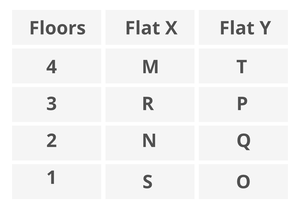Q9. Who among the following lives in Flat Y on the 4th floor?

(a) Q

(b) P

(c) M

(d) T

(e) None of these

Solution: d

T lives in Flat Y on the 4th floor.

Q10. Who among the following lives in the west of O?

(a) N

(b) S

(c) Q

(d) R

(e) None of these

Solution: b

S lives in the west of O.

Q11. Who among the following lives just below P’s Flat?

(a) N

(b) O

(c) Q

(d) T

(e) None of these

Solution: c

Q lives just below P’s Flat.

Q12. Four among the following five are alike in a certain way and related to a group, who among the following does not belong to the group?

(a) Q

(b) N

(c) R

(d) S

(e) M

Solution: a

Q does not belong to the group.

Q13. Which of the following statement is true about R?

(a) R lives on an odd-numbered floor

(b) T lives above R

(c) O lives south-east of R

(d) R and S live in the same flat.

(e) All are true

Solution: e

All the statement is true.

Directions (14-18): Answer these questions referring to the letter sequence given below:

### J Z H I X Y A N O F T W C K G U V E M P B L Q R D S

Q14. If the letters of the above-given series are written in reverse order, which letter will be the fifth to the left of the eleventh letter from the right end?

(a) O

(b) W

(c) T

(d) N

(e) U

Solution: e

After writing in reverse order, U will be the fifth to the left of eleventh letter from the right end.

Q15. What will come in place of question mark (?) in the following series?

ZXI  NTF  KVU  ?

(a) XAN

(b) NWT

(c) GMP

(d) BRQ

(e) None of these

Solution: e

PQL will come in place of the question mark.

Q16. Which of the following is 4th to the right of the 15th letter from the left end?

(a) M

(b) P

(c) N

(d) B

(e) None of these

Solution: a

M is 4th to the right of the 15th letter from the left end.

Q17. How many consonants are there which are immediately preceded by a consonant and immediately followed by a vowel?

(a) One

(b) Two

(c) Three

(d) Four

(e) None

Solution: c

Three consonants are immediately preceded by a consonant and immediately followed by a vowel.

Q18. If all vowels are eliminated, then which of the following 9th to the left of the element which is 8th from the right end?

(a) T

(b) X

(c) F

(d) Y

(e) None of these

Solution: d

Y will be 9th to the left of the element which is 8th from the right end.

Directions (19-22): Study the following information carefully and answer the questions given below.

Seven persons sit around a circular table facing the centre. Two persons sit between U and W. T and U are not an immediate neighbor. X sits 4th to the left of W. Y does not sit immediate right of Z. T sits immediate left of V.

Solution(19-22):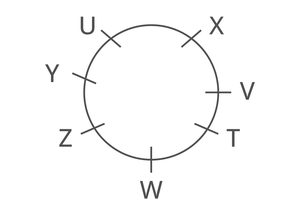Q19. Who sits 3rd to the right of Z?

(a) W

(b) T

(c) V

(d) U

(e) None of these

Solution: c

V sits 3rd to the right of  Z.

Q20. How many persons sit between X and Y when counted to the left of X?

(a) One

(b) Four

(c) Two

(d) Three

(e) Either (a) or (b)

Solution: b

Four persons sit between X and Y when counted to the left of X.

Q21. Who sits immediate right of U?

(a) Z

(b) Y

(c) W

(d) X

(e) None of these

Solution: b

Y sits immediate right of U.

Q22. The number of persons sit between Y and X, when counted to the left of Y is same as the number of persons sit between Z and ___, when counted to the left of __?

(a) T

(b) V

(c) X

(d) U

(e) None of these

Solution: a

When counted to the left of Y is the same as the number of persons sitting between Z and T, When counted to the left of T.

Q23. In the word ‘DESKTOP’, how many pairs of the letters have the same number of letters between them in both forward and backward direction in the word as in alphabetical series?a) None

(b) Two

(c) One

(d) Four

(e) Three

Solution: d

Four pairs in the word

Directions (24-26): In each of the questions below are given some statements followed by two conclusions. You have to take the given statements to be true even if they seem to be at variance with commonly known facts. Read all the conclusions and then decide which of the given conclusions logically follows from the given statements disregarding commonly known facts.

Q24. Statements:

All Bat is Rat.

Few Bat is Tiger.

No Pen is Tiger.

Conclusions:

I. All Tiger being Bat is a possibility.

II. Some Rat are Pen is a possibility.

(a) If only conclusion I follows.

(b) If only conclusion II follows.

(c) If either conclusion I or II follows.

(d) If neither conclusion I nor II follows.

(e) If both conclusions I and II follow.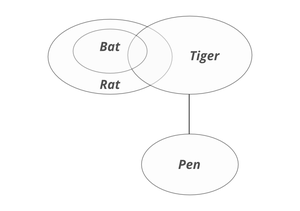Solution: e

both conclusions I and II follow.

Q25. Statements:

All Red are Pink.

No Green is Pink.

All Orange is Green.

Conclusions:

I. Some Orange are not Red.

II. Some Green are Red is a possibility.

(a) If only conclusion I follows.

(b) If only conclusion II follows.

(c) If either conclusion I or II follows.

(d) If neither conclusion I nor II follows.

(e) If both conclusions I and II follow.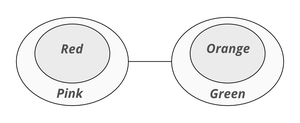Solution: a

If only conclusion I follows.

Q26. Statements:

Every Chair is Ball.

All Gun is Table.

Few Table are Chair.

Conclusions:

I. Some Ball is Gun.

II. No Ball is Gun.

(a) If only conclusion I follows.

(b) If only conclusion II follows.

(c) If either conclusion I or II follows.

(d) If neither conclusion I nor II follows.

(e) If both conclusions I and II follow.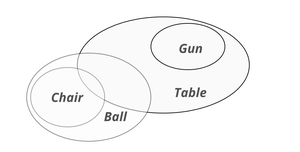Solution: c

If either conclusion I or II follows.

Q27. In a certain code TABLE is written as UZCKF in the same way how is MOUSE written in that code?

(a) NPTUF

(b) NNVRF

(c) NPTUD

(d) NNTUF

(e) None of these

Solution: b

MOUSE is coded NNVRF.

#### Directions (28-31): Study the following information and answer the questions below:

Seven persons were born in seven different months, i.e. January, March, April, May, September, October and December, of the same year and on the same date. Two persons were born between D and B. F is just older to C but not born in the month which has an even number of days. B was born in the month which has an even number of days. More than two persons were born between C and G, who is younger to D. A is older than E, who was not born in September.

Solution(28-31):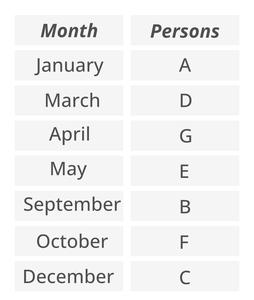Q28. Who among the following is the oldest person?

(a) D

(b) A

(c) G

(d) C

(e) None of these

Solution: b

A is the oldest person.

Q29. How many persons are younger than E?

(a) Four

(b) One

(c) Three

(d) Two

(e) None

Solution: c

Three persons are younger than E.

Q30. Who among the following was born in April?

(a) A

(b) E

(c) F

(d) G

(e) None of these

Solution: d

G was born in April.

Q31. Which of the following statement is not true about G?

(a) B is older than D

(b) G was born in April

(c) Four persons were born after G

(d) E is just younger than G

(e) Both (a) and (d)

Solution: a

B is older than D.

#### Directions (32-34): Read the following information carefully and answer the given questions:

Sonali starts walking from point S in north direction after walking 12km she reached at point R then she takes a right turn and covers 8km after that she takes a left turn and covers 6km to reach at point N. Finally, she takes a left turn and walks 10km to reach at point M.

Solution(32-34):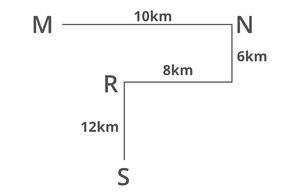Q32. If point L is 6km south of point M then what is the shortest distance between point R and point L?

(a) 5km

(b) 7km

(c) 2km

(d) 4km

(e) None of these

Solution: c

2km is the shortest distance between R and L.

Q33. Find out the shortest distance between point R and point N?

(a) 8km

(b) 9km

(c) 7km

(d) 10km

(e) None of these

Solution: d

10km shortest distance between R and N.

Q34. What is the direction of point M with respect to point S?

(a) North-East

(b) North-West

(c) South-East

(d) South-West

(e) None of these

Solution: b

Point M is North-West with respect to point S.

Q35. In the given word “MONSTER” if all the consonants are replaced with its next letter and all the vowels replaced with its previous letter after that how many letters are repeated in the new arrangement?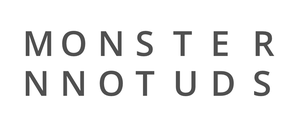(a) Three

(b) Four

(c) Two

(d) None

(e) One

Solution: e

One letter repeated in the new arrangement.

Whether you're preparing for your first job interview or aiming to upskill in this ever-evolving tech landscape, GeeksforGeeks Courses are your key to success. We provide top-quality content at affordable prices, all geared towards accelerating your growth in a time-bound manner. Join the millions we've already empowered, and we're here to do the same for you. Don't miss out - check it out now!

Previous
Next